# How To Find Y Parameters of Two Port Network (Examples)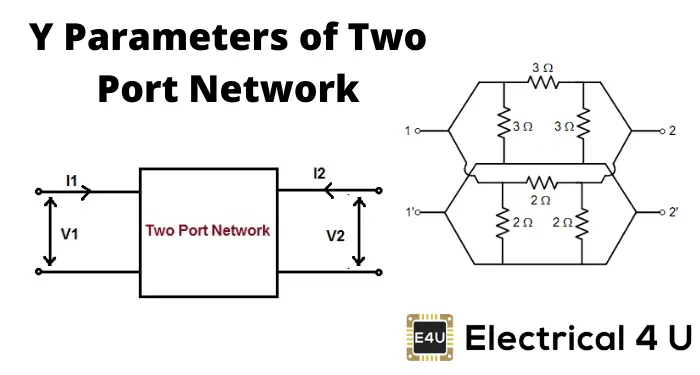## What is a Y Parameter?

Y parameters (also known as admittance parameters or short-circuit parameters) are properties used in electrical engineering to describe the electrical behavior of linear electrical networks. These Y-parameters are used in Y-matrixes (admittance matrixes) to calculate the incoming and outgoing voltages and currents of a network.

Y-parameters are also known as “short-circuit impedance parameters”, as they are calculated under open-circuit conditions. That is to say that Ix=∞, where x=1, 2 refers to the input and output currents flowing through the ports of a two port network.

Y parameters are commonly used alongside Z parameters, h parameters, and ABCD parameters to model and analyze transmission lines.

## How to Find Y Parameters in Two Port Networks

The below example goes over how to calculate the Y parameters of a two-port network. Note that Y parameters are also known as admittance parameters, and these terms may be used interchangeably in these examples.

When analyzing Z parameters (also known as impedance parameters), we express voltage in the term of current by the following equations.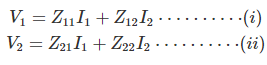Similarly, we can represent current in terms of voltage bt admittance parameters of a two port network. Then we will represent the current-voltage relations as,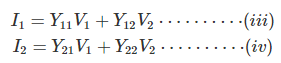This can also be represented in matrix form as,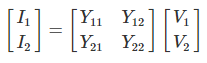Here, Y11, Y12, Y21, and Y22 are admittance parameters (or Y parameters).
We can determine the values of the parameters of a particular two port network by making a short-circuited output port and input port alternatively as follows.
First, let us apply a current source of I1 at the input port keeping the output port short-circuited as shown below.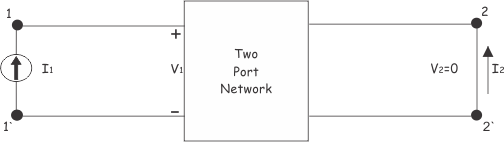In this case, the voltage across the output port will be zero since the terminals of the port are short-circuited.

Now, the ratio of input current I1 to input voltage V1 while output voltage V2 = 0, is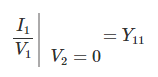This is called short circuit input admittance.
The ratio of output current I2 to input voltage V1 while output voltage V2 = 0, is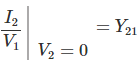This is referred to as the short circuit transfer admittance from the input port to the output port.
Now, let us short circuit the input port of the network and apply current I2 at the output port, as shown below.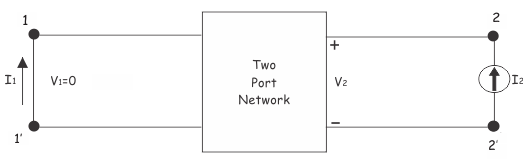In this case,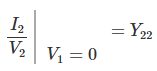This is called short circuit output admittance.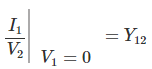This is called short circuit transfer admittance from the input port to the output port.
So finally,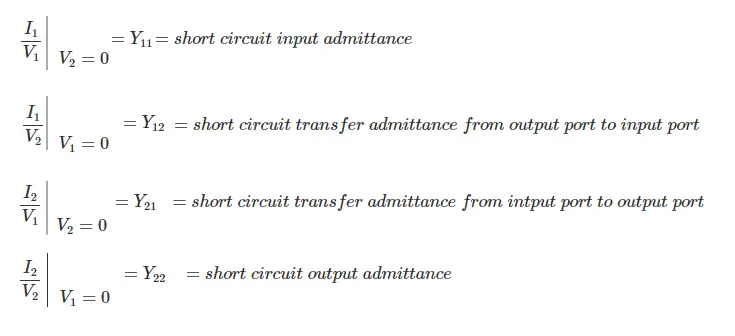Want To Learn Faster? 🎓
Get electrical articles delivered to your inbox every week.
No credit card required—it’s 100% free.